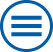# Research Groups

Partial Differential Equations
The theoretical understanding of differential equations, the modeling of real-world processes, the development of efficient numerical algorithms along with the new convergence methods and suitable hybrid models for multiscale problems are in the focus of the research group. The current applications embrace the mathematical modeling of charge-carrier transport in semiconductor materials on a variety of time and length scales, of general reaction-diffusion processes and of nonlinear material behavior.
Laser Dynamics
The research of this group is devoted to the mathematical modeling, as well as to the theoretical and numerical analysis of nonlinear dynamical processes, that emerge in optical technologies. The investigations are focused on dynamics of semiconductor lasers, pulses in nonlinear optical media and theory of dynamical systems. Typical mathematical questions concern structural properties of the models, their problem-specific reduction, as well as the dependence of nonlinear effects on design- and control parameters.
Numerical Mathematics and Scientific Computing
Main fields of activity of the group are the development and investigation of numerical methods for the solution of partial differential equations and algebro-differential equations for solving problems from application areas like simulation of semiconductors and electrochemical systems or optimization problems with partial differential equations. For this purpose, scientific software packages are developed and maintained.
Nonlinear Optimization and Inverse Problems
The group investigates nonlinear optimization and inverse problems occurring in current engineering and economic applications. A specific focus of research in optimization and optimal control is the investigation of special problems resulting from the presence of uncertain and nonsmooth data. Research in inverse problems is centered around the reconstruction of geometries and surfaces with complex or stochastic components from the scattering of acoustic, electromagnetic, or elastic waves.
Interacting Random Systems
The group analyses interacting random systems with many degrees of freedom. Examples come from statistical physics, telecommunication, population genetics, and materials science. Interactions are defined microscopically, and the macroscopic or mesoscopic behaviour is described using methods from probability theory (ergodic theorems, large deviations, martingale theory, weak convergence of measures,) and analysis (variational analysis, spectral theory, partial differential equations, ...).
Stochastic Algorithms and Nonparametric Statistics